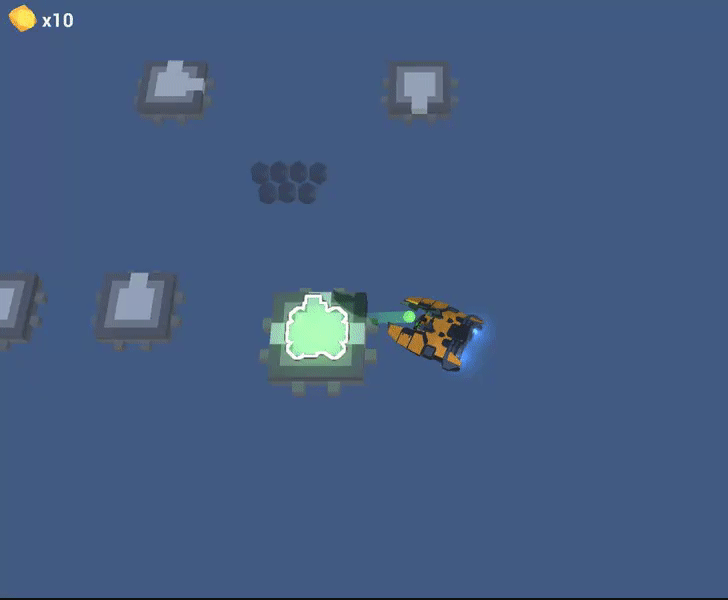# Buying stuff like in Kingdom: New Lands.

I implemented this technique in my game. Feel free to use it. The idea of it is that you see the “price” nodes above the building you want to buy.``````
[SerializeField]
private int currentPrice = 0; // how many nodes should be used in price. I made up to 12.
[SerializeField]
private float size = 1.0f;
[SerializeField]
private Transform[] nodeObjs; // predefined price nodes
[SerializeField]
private float arcSize = 0.1f; // the height of the arc. ( showing row like parabola )
[SerializeField]
[SerializeField]
private Vector3 offset = new Vector3(0,36,0);
[SerializeField]
private List<Vector3> positions = new List<Vector3>(); // position array of used nodes

/// <summary>
/// Bad in math. Good in bed.
/// </summary>
void CalculateNodes()
{
positions.Clear();
// Add nodes to array with we will work
Transform[] nodes = new Transform[currentPrice];
for (int i = 0;i<nodeObjs.Length;i++)
{
nodeObjs[i].gameObject.SetActive((i<currentPrice)?true:false);
if (currentPrice>i)
nodes[i] = nodeObjs[i];
}

// we will calculate single row length based on amount of price nodes.

int rowLength = currentPrice / 2;

if (currentPrice < 7)
rowLength = currentPrice;

float zPos = 0;
float extraOffset = 0;

//dirty hacks to be sure we can get nodes from the upper left corner.
List<Vector3> row1 = new List<Vector3>();
List<Vector3> row2 = new List<Vector3>();

for (int i = 0; i < currentPrice; i++)
{

float offset = 0;
float arcRatio = 0;
float yHeight = 0;

if (i < rowLength)
{
zPos = size * 1.25f;
extraOffset = 0;
if ((rowLength % 2) == 0)
extraOffset = size * 0.5f;
offset = (rowLength / 2 * size - extraOffset) * -1;
arcRatio = Mathf.PingPong((float)i / (float)(rowLength-1), 0.5f);

}
else
{
zPos = 0;
extraOffset = 0;
if (((currentPrice - rowLength) % 2) == 0)
extraOffset = size * 0.5f;
arcRatio = Mathf.PingPong((float)(i-rowLength) / (float)(currentPrice-rowLength-1), 0.5f);
offset = ((rowLength*size) + ((currentPrice-rowLength) / 2 * size - extraOffset)) * -1 ;
}
yHeight = Mathf.Sin(arcRatio * Mathf.PI)*arcSize;

nodes[i].localPosition = new Vector3(offset + i * size, yHeight, -zPos);
if (i < rowLength)
else

}
// Adding rows to positions array. You will need it to get price point index.

}
``````

Follow me at my Twitch channel where I’m showing creation process of the Battlecruiser.

Tags:

Categories:

Updated: